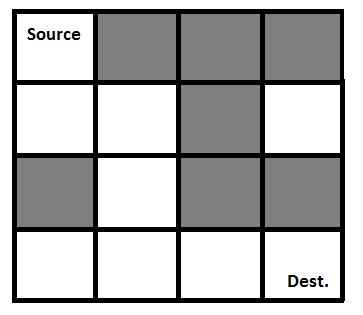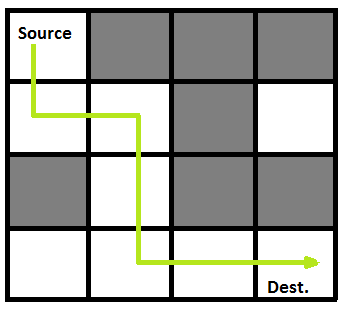Related Articles

# Python Program for Rat in a Maze | Backtracking-2

• Last Updated : 02 Aug, 2021

We have discussed Backtracking and Knight’s tour problem in Set 1. Let us discuss Rat in a Maze as another example problem that can be solved using Backtracking.

A Maze is given as N*N binary matrix of blocks where source block is the upper left most block i.e., maze and destination block is lower rightmost block i.e., maze[N-1][N-1]. A rat starts from source and has to reach the destination. The rat can move only in two directions: forward and down.
In the maze matrix, 0 means the block is a dead end and 1 means the block can be used in the path from source to destination. Note that this is a simple version of the typical Maze problem. For example, a more complex version can be that the rat can move in 4 directions and a more complex version can be with a limited number of moves.

Following is an example maze.

` Gray blocks are dead ends (value = 0). `Following is binary matrix representation of the above maze.

```                {1, 0, 0, 0}
{1, 1, 0, 1}
{0, 1, 0, 0}
{1, 1, 1, 1}
```

Following is a maze with highlighted solution path.Following is the solution matrix (output of program) for the above input matrix.

```                {1, 0, 0, 0}
{1, 1, 0, 0}
{0, 1, 0, 0}
{0, 1, 1, 1}
All entries in solution path are marked as 1.
```

## Python3

 `# Python3 program to solve Rat in a Maze``# problem using backtracking` `# Maze size``N ``=` `4` `# A utility function to print solution matrix sol``def` `printSolution( sol ):``    ` `    ``for` `i ``in` `sol:``        ``for` `j ``in` `i:``            ``print``(``str``(j) ``+` `" "``, end ``=``"")``        ``print``("")` `# A utility function to check if x, y is valid``# index for N * N Maze``def` `isSafe( maze, x, y ):``    ` `    ``if` `x >``=` `0` `and` `x < N ``and` `y >``=` `0` `and` `y < N ``and` `maze[x][y] ``=``=` `1``:``        ``return` `True``    ` `    ``return` `False` `""" This function solves the Maze problem using Backtracking.``    ``It mainly uses solveMazeUtil() to solve the problem. It``    ``returns false if no path is possible, otherwise return``    ``true and prints the path in the form of 1s. Please note``    ``that there may be more than one solutions, this function``    ``prints one of the feasable solutions. """``def` `solveMaze( maze ):``    ` `    ``# Creating a 4 * 4 2-D list``    ``sol ``=` `[ [ ``0` `for` `j ``in` `range``(``4``) ] ``for` `i ``in` `range``(``4``) ]``    ` `    ``if` `solveMazeUtil(maze, ``0``, ``0``, sol) ``=``=` `False``:``        ``print``(``"Solution doesn't exist"``);``        ``return` `False``    ` `    ``printSolution(sol)``    ``return` `True``    ` `# A recursive utility function to solve Maze problem``def` `solveMazeUtil(maze, x, y, sol):``    ` `    ``# if (x, y is goal) return True``    ``if` `x ``=``=` `N ``-` `1` `and` `y ``=``=` `N ``-` `1``:``        ``sol[x][y] ``=` `1``        ``return` `True``        ` `    ``# Check if maze[x][y] is valid``    ``if` `isSafe(maze, x, y) ``=``=` `True``:``        ``# mark x, y as part of solution path``        ``sol[x][y] ``=` `1``        ` `        ``# Move forward in x direction``        ``if` `solveMazeUtil(maze, x ``+` `1``, y, sol) ``=``=` `True``:``            ``return` `True``            ` `        ``# If moving in x direction doesn't give solution``        ``# then Move down in y direction``        ``if` `solveMazeUtil(maze, x, y ``+` `1``, sol) ``=``=` `True``:``            ``return` `True``        ` `        ``# If none of the above movements work then``        ``# BACKTRACK: unmark x, y as part of solution path``        ``sol[x][y] ``=` `0``        ``return` `False` `# Driver program to test above function``if` `__name__ ``=``=` `"__main__"``:``    ``# Initialising the maze``    ``maze ``=` `[ [``1``, ``0``, ``0``, ``0``],``             ``[``1``, ``1``, ``0``, ``1``],``             ``[``0``, ``1``, ``0``, ``0``],``             ``[``1``, ``1``, ``1``, ``1``] ]``             ` `    ``solveMaze(maze)` `# This code is contributed by Shiv Shankar`
Output:
```1 0 0 0
1 1 0 0
0 1 0 0
0 1 1 1
```

Please refer complete article on Rat in a Maze | Backtracking-2 for more details!

My Personal Notes arrow_drop_up